# MCAT Physical : Other Fluid Principles

## Example Questions

### Example Question #21 : Fluids And Gases

Boiling point is the temperature a liquid needs to achieve in order to begin its transformation into a gaseous state. Campers and hikers who prepare food during their trips have to account for differences in atmospheric pressure as they ascend in elevation. During the ascent, the decrease in atmospheric pressure changes the temperature at which water boils.

Further complicating the matter is the observation that addition of a solute to a pure liquid also changes the boiling point. Raoult’s Law can be used to understand the changes in boiling point if a non-volatile solute is present, as expressed here.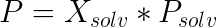In this law,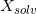is the mole fraction of the solvent,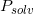is the vapor pressure of the pure solvent, andis the vapor pressure of the solution. When this vapor pressure is equal to the local atmospheric pressure, the solution boils.

Like boiling point change, osmotic pressure is a property influenced by the addition of solute to solvent. A scientist is studying a solution in a beaker. Osmotic pressure in this solution pulls __________ from __________ solute concentration to __________ solute concentration.

water . . . low . . . high

solute . . . low . . . high

solute . . . high . . . low

water . . . high . . . low

Osmotic pressure only exists across cell membranes

water . . . low . . . high

Explanation:

Osmotic pressure pulls water, as a pure solvent, into regions of high solute density to even out the solute concentration between different regions of solution.

Osmotic pressure is related to solute concentration, but does not affect the flow of solute.

### Example Question #22 : Fluids And Gases

The law of Laplace states what relationship between that the tension in the wall of a bubble and the radius of the bubble?

Tension is directly proportional to the square of the radius

Tension is inversely proportional to the square of the radius

Tension is inversely proportional to the radius

Tension is directly proportional to the radius

Tension is proportional to the cube of the radius

Tension is directly proportional to the radius

Explanation:

The law of Laplace states that wall tension in a bubble, balloon, pulmonary alveolus, or blood vessel is directly proportional to the radius and the internal pressure.

Law of Laplace: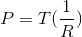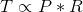In other words, as the size of the bubble increases at constant pressure or as the pressure increases at constant size, the wall tension needed to keep the bubble from increasing in size is increased. In part, this explains why aneurysms on blood vessels burst.

### Example Question #461 : Mcat Physical Sciences

Which of the following is a possible unit of measurement for surface tension?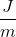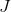Explanation:

Surface tension is a phenomenon observed in fluids, and is defined as the resistance of a fluid to outside force (or a resistance to increased surface area). Surface tension is measured in force per unit length.

The SI unit for force is Newtons and the SI unit for length is meters; therefore, the surface tension is usually measured as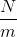.

Surface tension can also be measured in energy per unit area. Energy is measured in Joules and area is measured in square meters; therefore, the units for surface tension can also be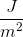.

We can see that these units are equivalent by looking at the conversion of Joules and Newtons: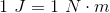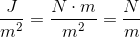### Example Question #462 : Mcat Physical Sciences

Which of the following is false regarding surface tension?

The interaction between molecules at the surface is stronger than the interaction between molecules below the surface

Surface tension prevents water molecules in a droplet from spreading

Surface tension forces water droplets to form spheres because they has the lowest surface area to volume ratio

Compared to an object that is completely submerged in water, an object floating on water has less resistance to motion

Compared to an object that is completely submerged in water, an object floating on water has less resistance to motion

Explanation:

Consider a beaker containing water. The molecules at the surface of water interact with fewer molecules than the molecules at the center of the beaker. The surface molecules have no water molecules above them, whereas the molecules in the center of the solution interact with molecules in all directions. Since the surface molecules have fewer interactions, the interactions between these molecules are much stronger than the interactions of a center molecule; fewer interactions have opposing counterparts, resulting in stronger forces.

Recall that surface tension can be defined as the resistance to increasing surface area. This means that the surface area is minimized due to surface tension. Of all the geometrical shapes, spheres have the lowest surface area to volume ratio; therefore, surface tension forces water droplets to take spherical shapes. Surface tension occurs due to the intermolecular forces between liquid molecules. These intermolecular forces between molecules are cohesive, preventing the spreading of water molecules.

It is harder to move an object that is floating on water than an object submerged in water. This occurs because the interactions between the molecules at the surface are stronger than the interactions below the surface. Moving an object involves moving the water molecules around it. Since it is harder to move molecules at the surface (stronger interactions), it is harder to move an object at the surface. An object floating on water will have more resistance to motion.

### Example Question #463 : Mcat Physical Sciences

Increased surface tension __________ the surface area of the molecule because it __________ the amount of molecules at the surface.

increases . . . decreases

decreases . . . decreases

increases . . . increases

decreases . . . increases